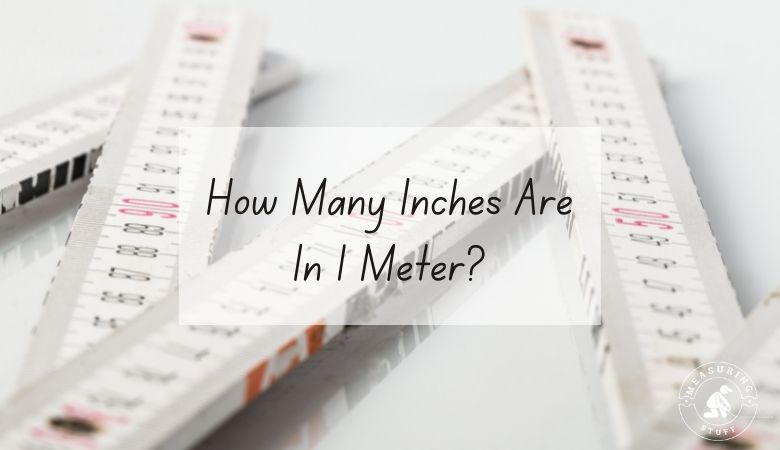# How Many Inches Are In 1 Meter?

Meters and inches are units of measurement for distance, height, or length.

Meters are used in the metric system, while inches are part of the imperial system.

Because they’re part of two different systems, it can sometimes be confusing to try to convert between the two.

A simple mathematical equation, however, can help.

There are about 39.37 inches in a single meter.

This means that if we want to figure out how many inches can be found in a given number of meters, we can multiply the number of meters by 39.37.

1 meter x 39.37 (conversion factor) = 39.37 inches.

For example, if you have 9 meters and you want to know how many inches are in those meters, you would multiply 9 by 39.37.

The answer is 354.33, which tells us that there are about 354 inches in 9 meters.

You can reverse this equation to figure out how many meters you can make out of a given number of inches.

To do this, you would divide the number of inches by 39.37.

For example, if you have 100 inches and you need to determine how many meters that is, you would need to divide 100 by 39.37.

With this result, we can see that there are about 2 and a half meters in 100 inches.

If you’re not comfortable doing the math or you need a faster answer, you can use a conversion chart.

## Meters into inches conversion table

You can also use our online conversion calculator below.

## Meters into inches conversion calculator

Use the following calculator to easily convert meters into inches. Simply type in the desired value and select meters in the convert from box and inches in the convert to box. The calculator will instantly do the math for you.

You can also convert 1 meter into other units of measurements.

• 1 meters = 3.281 feet
• 1 meters = 100 centimeters
• 1 meters = 1000 millimeters

Other articles on our site using meters and inches include:

## Meters and inches measurements

Metric measuring units use a base-ten system.

This makes it simple to figure out how many millimeters are in a meter or how many meters are in a kilometer.

The metric system originated in France, but today it’s popular around the world due to its simplicity.

It’s also widely used in scientific and medical fields thanks to its accuracy and ease of conversion.

Inches are part of the standard imperial system.

This system is commonly used in the US, although it’s sometimes used in other parts of the world.

The inch measurement was originally based on the length of an adult person’s thumb.

These thumb lengths were used to break up the foot measurement, which was based, as the name suggests on the length of an adult’s foot.

Generally, there were 10 or 12 of these thumb lengths or inches in a foot, and this is still how we divide a standard foot today.

You can still use your thumb to estimate lengths, as the average adult’s thumb is about 1 inch long.